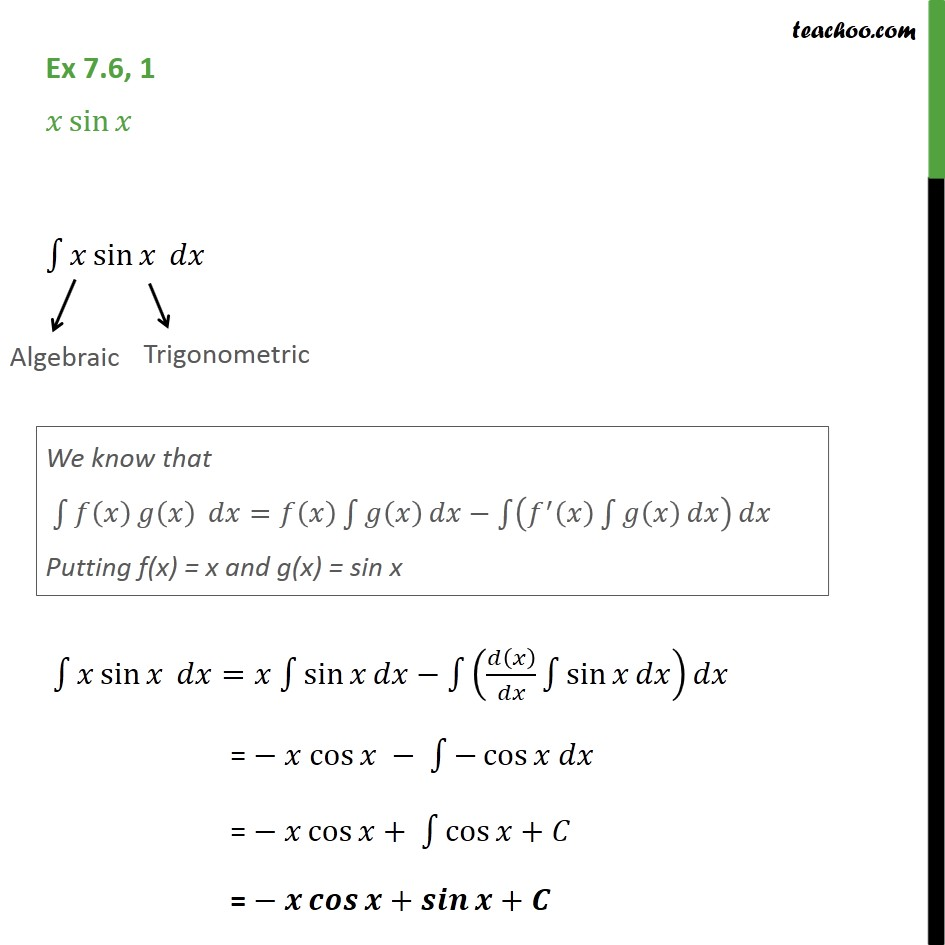1. Chapter 7 Class 12 Integrals (Term 2)
2. Serial order wise
3. Ex 7.6

Transcript

Ex 7.6, 1 - Chapter 7 Class 12 Integration - NCERT Book Integrate x sin x ∫ x sin x dx | | Algebraic Trigonometric We solve this by Integration by parts We know that ∫ f(x) g(x) dx = f(x) ∫𝑔(𝑥) 𝑑𝑥 − ∫(𝑓′ (𝑥) ∫ 𝑔(𝑥) 𝑑𝑥) 𝑑𝑥 Putting f(x) = x and g(x) = sin x ∫1▒〖𝑥 sin⁡𝑥 〗 𝑑𝑥=𝑥 ∫1▒sin⁡𝑥 𝑑𝑥−∫1▒(𝑑(𝑥)/𝑑𝑥 ∫1▒〖sin⁡𝑥 𝑑𝑥〗) 𝑑𝑥 = − 𝑥 cos⁡𝑥 − ∫1▒〖−cos⁡〖𝑥 𝑑𝑥〗 〗 = − 𝑥 cos⁡𝑥+ ∫1▒〖cos⁡𝑥+𝐶〗 = − 𝒙 𝒄𝒐𝒔⁡𝒙+𝒔𝒊𝒏⁡𝒙+𝑪

Ex 7.6